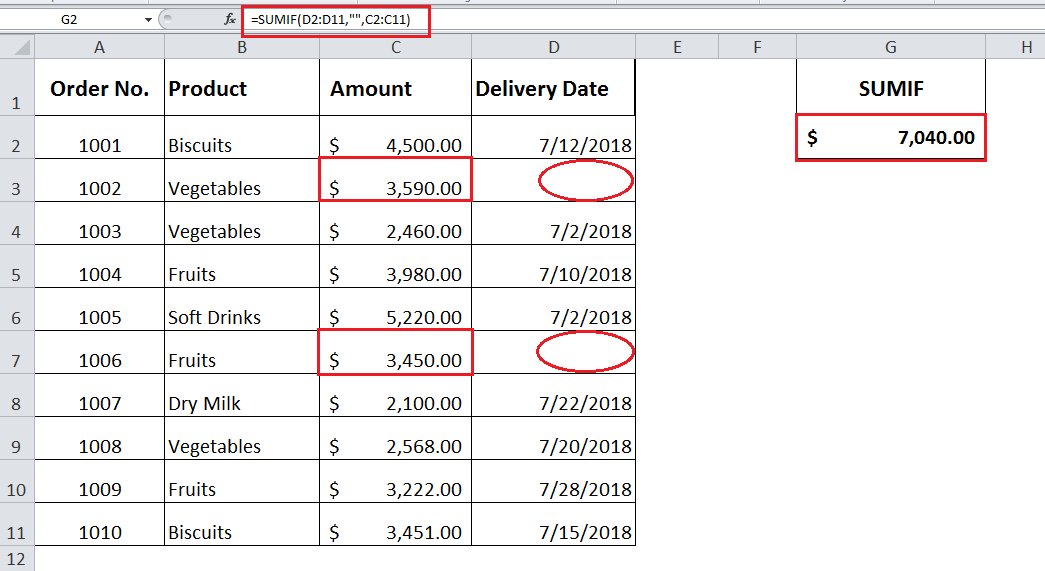Get instant live expert help with Excel or Google Sheets“My Excelchat expert helped me in less than 20 minutes, saving me what would have been 5 hours of work!”

#### Post your problem and you’ll get expert help in seconds.

Your message must be at least 40 characters
Our professional experts are available now. Your privacy is guaranteed.

# Add Cells Based on Blank / Not Blank Criteria

Using Excel SUMIF function you can sum the numbers from a range of cells that meet the criteria based on cells that do not contain any value (Blank) or cells that are contain a value (Not Blank).

While working with data you come across to a situation where you need to sum numbers based on these two criteria. Each of these criteria can be tested on data range or sum_range as per SUMIF syntax.

`=SUMIF (range, criteria, sum_range)`

In this article, suppose you have a data set of sales orders of various products with their amounts and delivery dates, and some of the dates are missing (Blank) and some are delivery dates are provided (Not Blank) in Delivery Date field.

### SUMIF Blank cells

Here, you will learn how to sum numbers based on blank cells criteria. In this example you need to sum Amounts where delivery dates are not mentioned in Delivery Date field. Criterion is supplied as double quotation marks without any space in-between, such as “”.

`=SUMIF(D2:D11,"",C2:C11)`

This criteria implies to all those cells that contain zero character length, means empty. If a cell contains any space, then it will not meet criteria and it will be considered as Non Blank cell.

This formula will sum all those amounts in range C2:C11 where cells are Blank in range D2:D11.Here, you can see SUMIF function has sum the numbers (Amount) from range specified as sum_range, where cells in specified range do not contain a value (Delivery Date).

### SUMIF Non Blank cells

Using SUMIF function you can sum numbers based on Non blank cells criteria. In our examples, you need to sum Amounts where delivery dates are mentioned in Delivery Date field.

Criteria in SUMIF function is supplied by using one of comparison operators Not Equal to (<>), means not equal to blank, in double quotation marks, such as “<>”.

`=SUMIF(D2:D11,"<>",C2:C11)`

This formula will sum all those amounts in range C2:C11 where cells are Not Blank in range D2:D11. Criteria implies to all those cells that contain at least one character length, means not empty. If a cell contains even a space, then it will meet criteria and will be considered as Non Blank cell.SUMIF function will sum the numbers (Amount) in specified sum_range where criteria is met for Non Blank cells in Delivery Date field, specified as range as per syntax.

### Did this post not answer your question? Get a solution from connecting with the expert.Another blog reader asked this question today on Excelchat:
Related blogs
Solution examplesamending a formula so that there is another criteria and if it does not meet this criteria then we stick with the original criteria
Solved by A. B. in 40 minsI need Column D to = Column C - Column A only if Column B has a 'Date' entered.
Solved by I. B. in 11 minsHello, I need help trying to create a sum of numerical values on a spreadsheet in which the only values that apply to the sum are when the status of another cell is a certain value (e.g. value is not in sum when status: closed, value is in sum when status: open)
Solved by T. W. in 18 minsI am looking for a formula to SUM column L, if Column A matched the relevant date and column C matches the name
Solved by Z. Y. in 16 minsI need a formula that is essentially saying IF this range of cells contain a certain name, add the number in another cell on the same row, and them sum that number in a different cell..!
Solved by O. H. in 44 mins## Subscribe to Excelchat.coAnother blog reader asked this question today on Excelchat: